# Regularized Logistic regression

Previously we have tried logistic regression without regularization and with simple training data set. Bu as we all know, things in real life aren’t as simple as we would want to. There are many types of data available the need to be classified. Number of features can grow up hundreds and thousands while number of instances may be limited. Also in many times we might need to classify in more than two classes.

The first problem that might rise due to large number of features is over-fitting. This is when learned hypothesis hΘ (x) fit training data too well (cost J(Θ) 0), but it fails when classifying new data samples. In other words, model tries to distinct each training example correctly by drawing very complicated decision boundary between training data points.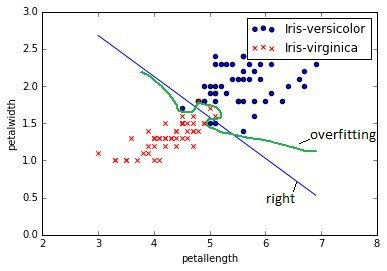As you can see in image above, over-fitting would be green decision boundary. So how to deal with over-fitting problem? There might be several approaches:

• Reducing number of features;
• Selecting different classification model;
• Use regularization.

We leave first two out of question, because selecting optimal number of features is a different topic of optimization. Also we are sticking with logistic regression model for now, so changing classifier is also out of question. We choose third option which is more general and proper way of addressing problem.

## Regularized cost function and gradient descent

In order to regularize cost function we need to add penalization to it. Mathematically speaking we need to add norm of parameter vector multiplied with regularization parameter λ. By selecting regularization parameter, we can fine tune the fitting.

This is cost function with regularization: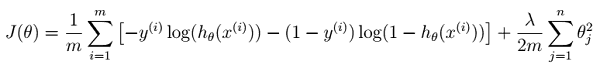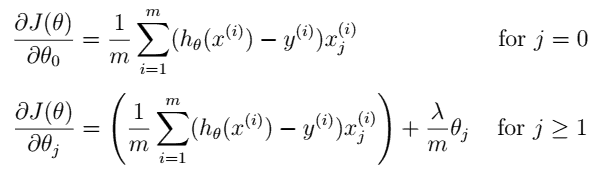We can see that parameter Θ0 should now be regularized.

The other procedure remains same – we need to minimize J(Θ) until converge.

Python code of cost function:

```def cost(theta, X, y, lmd):
'''  regularized logistic regression cost    '''
sxt = sigmoid(np.dot(X, theta));
mcost = (-y)*np.log(sxt) - (1-y)*np.log(1-sxt) + lmd/2 * np.dot(theta.T,theta);
return mcost.mean()```

Feature normalization

A good practice is to normalize training data in order to bring scales to similar level. This makes optimization task more easier and less intense. For manual operations you could use mean normalization (sometimes called Z score):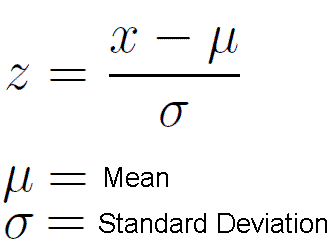If you don’t want to waste time coding, then use scale function from scikit-learn library. As you progress further, you will find that all tools and functions required for most of machine learning tasks can be find in this library.

```from sklearn import svm, preprocessing
#...
XN = preprocessing.scale(X);```

For optimization of Θ we are going to use function called fmin_bfgs which takes cost function, initial thetas and produce thetas of minimized function. This is same as previously, the only new thing is lambda parameter which should be included in to parameter list.

```#create initial theta values
theta = 0.1* np.random.randn(n);
#initial lambda
lmd = 0.005;
#use fmin_bfgs optimisation function find thetas
theta = opt.fmin_bfgs(cost, theta, args=(XX, Y, lmd));```

### Evaluating logistic regression classifier with regularization

This time we are going to use more complex data set downloaded from kaggle.com. Data set consists of 569 instances having 30 features. Features are calculated from real cell nucleus digitized images taken from breast mass using fine needle aspirate (FNA).

a) radius (mean of distances from center to points on the perimeter) b) texture (standard deviation of gray-scale values) c) perimeter d) area e) smoothness (local variation in radius lengths) f) compactness (perimeter^2 / area – 1.0) g) concavity (severity of concave portions of the contour) h) concave points (number of concave portions of the contour) i) symmetry j) fractal dimension (“coastline approximation” – 1)

It is hard to plot data set in one plot, this might be done by plotting plot matrix where each pair of features might be represented. Also this could be done with 3D graphs. This is a fragment of plot matrix: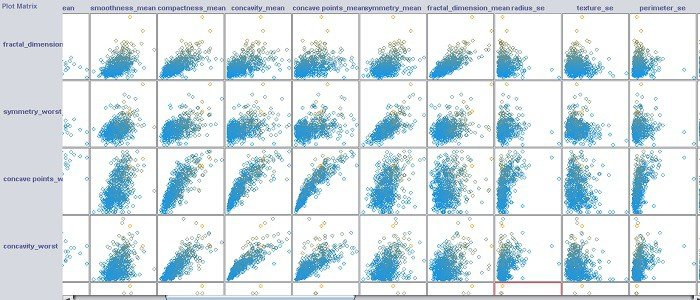Each patient has marker either it is malignant or benign. In order to teach classifier and test it, we split database in to two sets – training set and test set. By applying test set to built model we can validate if model is good.

Running model with test set we get Accuracy: 98.1%.

Algorithm and data sets for your own try is here: logistic_regression_reg